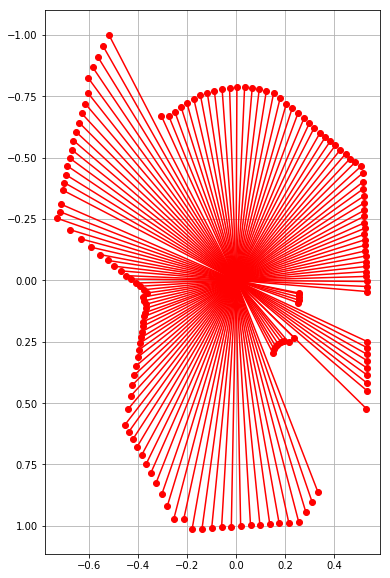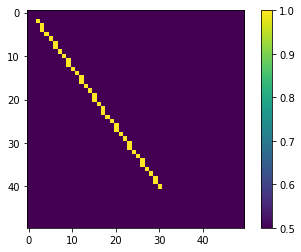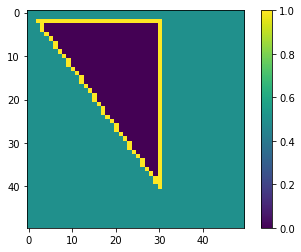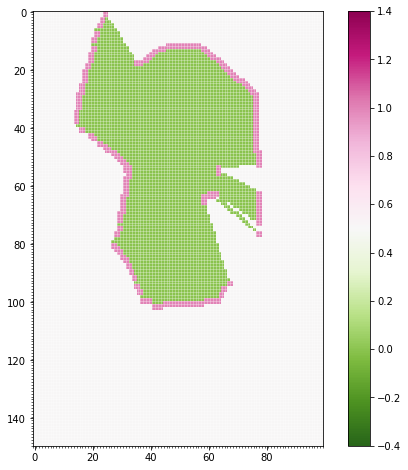# Lidar to grid mapThis simple tutorial shows how to read LIDAR (range) measurements from a file and convert it to occupancy grid.

Occupancy grid maps (Hans Moravec, A.E. Elfes: High resolution maps from wide angle sonar, Proc. IEEE Int. Conf. Robotics Autom. (1985)) are a popular, probabilistic approach to represent the environment. The grid is basically discrete representation of the environment, which shows if a grid cell is occupied or not. Here the map is represented as a `numpy array`, and numbers close to 1 means the cell is occupied (marked with red on the next image), numbers close to 0 means they are free (marked with green). The grid has the ability to represent unknown (unobserved) areas, which are close to 0.5.

In order to construct the grid map from the measurement we need to discretise the values. But, first let’s need to `import` some necessary packages.

```import math
import numpy as np
import matplotlib.pyplot as plt
from math import cos, sin, radians, pi
```

The measurement file contains the distances and the corresponding angles in a `csv` (comma separated values) format. Let’s write the `file_read` method:

```def file_read(f):
"""
Reading LIDAR laser beams (angles and corresponding distance data)
"""
measures = [line.split(",") for line in open(f)]
angles = []
distances = []
for measure in measures:
angles.append(float(measure))
distances.append(float(measure))
angles = np.array(angles)
distances = np.array(distances)
return angles, distances
```

From the distances and the angles it is easy to determine the `x` and `y` coordinates with `sin` and `cos`. In order to display it `matplotlib.pyplot` (`plt`) is used.

```ang, dist = file_read("lidar01.csv")
ox = np.sin(ang) * dist
oy = np.cos(ang) * dist
plt.figure(figsize=(6,10))
plt.plot([oy, np.zeros(np.size(oy))], [ox, np.zeros(np.size(oy))], "ro-") # lines from 0,0 to the
plt.axis("equal")
bottom, top = plt.ylim()  # return the current ylim
plt.ylim((top, bottom)) # rescale y axis, to match the grid orientation
plt.grid(True)
plt.show()
```The `lidar_to_grid_map.py` contains handy functions which can used to convert a 2D range measurement to a grid map. For example the `bresenham` gives the a straight line between two points in a grid map. Let’s see how this works.

```import lidar_to_grid_map as lg
map1 = np.ones((50, 50)) * 0.5
line = lg.bresenham((2, 2), (40, 30))
for l in line:
map1[l][l] = 1
plt.imshow(map1)
plt.colorbar()
plt.show()
``````line = lg.bresenham((2, 30), (40, 30))
for l in line:
map1[l][l] = 1
line = lg.bresenham((2, 30), (2, 2))
for l in line:
map1[l][l] = 1
plt.imshow(map1)
plt.colorbar()
plt.show()
```To fill empty areas, a queue-based algorithm can be used that can be used on an initialized occupancy map. The center point is given: the algorithm checks for neighbour elements in each iteration, and stops expansion on obstacles and free boundaries.

```from collections import deque
def flood_fill(cpoint, pmap):
"""
cpoint: starting point (x,y) of fill
pmap: occupancy map generated from Bresenham ray-tracing
"""
# Fill empty areas with queue method
sx, sy = pmap.shape
fringe = deque()
fringe.appendleft(cpoint)
while fringe:
n = fringe.pop()
nx, ny = n
# West
if nx > 0:
if pmap[nx - 1, ny] == 0.5:
pmap[nx - 1, ny] = 0.0
fringe.appendleft((nx - 1, ny))
# East
if nx < sx - 1:
if pmap[nx + 1, ny] == 0.5:
pmap[nx + 1, ny] = 0.0
fringe.appendleft((nx + 1, ny))
# North
if ny > 0:
if pmap[nx, ny - 1] == 0.5:
pmap[nx, ny - 1] = 0.0
fringe.appendleft((nx, ny - 1))
# South
if ny < sy - 1:
if pmap[nx, ny + 1] == 0.5:
pmap[nx, ny + 1] = 0.0
fringe.appendleft((nx, ny + 1))
```

This algotihm will fill the area bounded by the yellow lines starting from a center point (e.g. (10, 20)) with zeros:

```flood_fill((10, 20), map1)
map_float = np.array(map1)/10.0
plt.imshow(map1)
plt.colorbar()
plt.show()
```Let’s use this flood fill on real data:

```xyreso = 0.02  # x-y grid resolution
ox = np.sin(ang) * dist
oy = np.cos(ang) * dist
pmap, minx, maxx, miny, maxy, xyreso = lg.generate_ray_casting_grid_map(ox, oy, xyreso, False)
xyres = np.array(pmap).shape
plt.figure(figsize=(20,8))
plt.subplot(122)
plt.imshow(pmap, cmap = "PiYG_r")
plt.clim(-0.4, 1.4)
plt.gca().set_xticks(np.arange(-.5, xyres, 1), minor = True)
plt.gca().set_yticks(np.arange(-.5, xyres, 1), minor = True)
plt.grid(True, which="minor", color="w", linewidth = .6, alpha = 0.5)
plt.colorbar()
plt.show()
```
```The grid map is  150 x 100 .
```